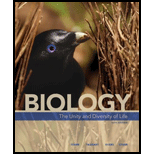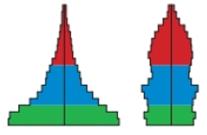Chapter 44, Problem 4CT### Biology: The Unity and Diversity o...

15th Edition
Cecie Starr + 3 others
ISBN: 9781337408332

#### Solutions

Chapter
Section### Biology: The Unity and Diversity o...

15th Edition
Cecie Starr + 3 others
ISBN: 9781337408332
Textbook Problem
10 views

# The age structure diagrams for two hypothetical populations are shown below. Describe the growth rate of each population and discuss the current and future social and economic problems that each is likely to face.Summary Introduction

To explain: The growth rate for the given population.

Concept introduction: The age structure of a population is the proportion of individuals in each of several age categories. Individuals, who are capable for reproducing, constitute a reproductive base of a population. Age structure affects the population’s growth rate. Age structure reflects the demographic and socio-economic history of a population over a period of time.

Explanation

The age structure diagram given is in pre-industrialized form with the intense growth rate. The age structure resembles a developing nation. The growth rate of this age structure is rapidly increasing. The broader the base of an age structure diagram, the greater is the population growth...

Summary Introduction

To explain: The social and economic problems faced by the given population in the future.

Concept introduction: The age structure of a population is the proportion of individuals in each of several age categories. Individuals, who are capable for reproducing, constitute a reproductive base of a population. Age structure affects a population’s growth rate. Age structure reflects the demographic and socio-economic history of a population over a period of time.

### Still sussing out bartleby?

Check out a sample textbook solution.

See a sample solution

#### The Solution to Your Study Problems

Bartleby provides explanations to thousands of textbook problems written by our experts, many with advanced degrees!

Get Started